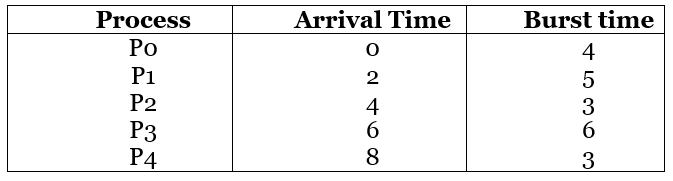# Highest Response Ratio Next (HRRN) Scheduling in Operating System

HRRN Scheduling in OS: In this tutorial, we will discuss the HRRN Scheduling Algorithm in Operating System. We will define it first and illustrate it with the help of an example and will understand its advantages and disadvantages.
Submitted by Monika Jha, on September 25, 2019

## What is HRRN Scheduling in Operating System?

• HRRN is the abbreviation of Highest Response Ratio Next Scheduling. It is an optimal scheduling algorithm.
• It is non-preemptive scheduling algorithm that means if there is any process currently executing with the CPU and if a new process arrives in the memory and its burst time is smaller than the currently running process then currently running process will not be put in the ready queue and it can complete its execution without any interruption.
• Non-preemptive scheduling algorithms are designed so that once a process starts its execution, it cannot be preempted until it completes its allotted time.
• It is the modification of shortest job next (SJN) to mitigate the problem of process starvation.
• In this scheduling algorithm assign the CPU to the next process which has the highest response ratio not according to the shortest burst time.

### Algorithm

• This scheduling is done based on the response ratio. Response ratio can be calculated with arrival time, waiting time and CPU burst time.
```    Response Ratio = (W+S)/S
Where, W= waiting time
S= Burst time ```
• In this scheduling, once a process selected for execution then it will run until its completion.
• First, we have to calculate the waiting time for all the processes. The sum of the periods spent waiting in the ready queue is referred to as waiting time.
• Each time processes get scheduled for execution to find response ratio for each available process.
• Process having shortest response ratio will be executed first by the processor.
• If two processes have the same response ratio then break the tie using the FCFS scheduling algorithm.

Example:

Here is an example of HRRN Scheduling given arrival time and burst or service time of each process,Solution:Explanation:

• At time t = 0, only the process P0 is available in the ready queue. So, process P0 executes till its completion.
• At time t = 4, only the process P1 and P2 are available in the ready queue. So, we have to calculate the response ratio.
• The process which has the highest response ratio will be executed next.
• Response Ratio are,
```    RR (P1) = [(4 - 3) + 3] / 3 = 1.40
RR (P2) = [(4 - 3) + 3] / 3 = 0.75```
• Process P1 has highest response ratio so it will selected for execution.
• After the completion of execution of process P1, there are three processes P2, P3 and P4 are in the ready queue.
• So, the Response Ratio for processes P2, P3 and P4 are,
```    RR (P2) = [(9 - 4) + 3] / 3 = 2.66
RR (P3) = [(9 - 6) + 6] / 6 = 1.50
RR (P4) = [(9 - 8) + 3] / 3 = 1.33```
• Process P2 has highest response ratio so it will selected for execution.
• After the completion of execution of process P2, there are three processes P3 and P4 are in the ready queue.
• So, the Response Ratio for processes P3 and P4 are,
```    RR (P3) = [(12 - 6) + 6] / 6 = 2
RR (P4) = [(12 - 8) + 3] / 3 = 2.33```
• Process P4 has highest response ratio so it will be executed next.
• After the completion of the execution of process P4, there are only process P3 in the ready queue. So, it will be executed next.

• Its performance is better than SJF Scheduling.
• It limits the waiting time of longer jobs and also supports shorter jobs.

• It can't be implemented practically.
• This is because the burst time of all the processes can not be known in advance.

What's New

Top Interview Coding Problems/Challenges!

Languages: » C » C++ » C++ STL » Java » Data Structure » C#.Net » Android » Kotlin » SQL
Web Technologies: » PHP » Python » JavaScript » CSS » Ajax » Node.js » Web programming/HTML
Solved programs: » C » C++ » DS » Java » C#
Aptitude que. & ans.: » C » C++ » Java » DBMS
Interview que. & ans.: » C » Embedded C » Java » SEO » HR
CS Subjects: » CS Basics » O.S. » Networks » DBMS » Embedded Systems » Cloud Computing
» Machine learning » CS Organizations » Linux » DOS
More: » Articles » Puzzles » News/Updates